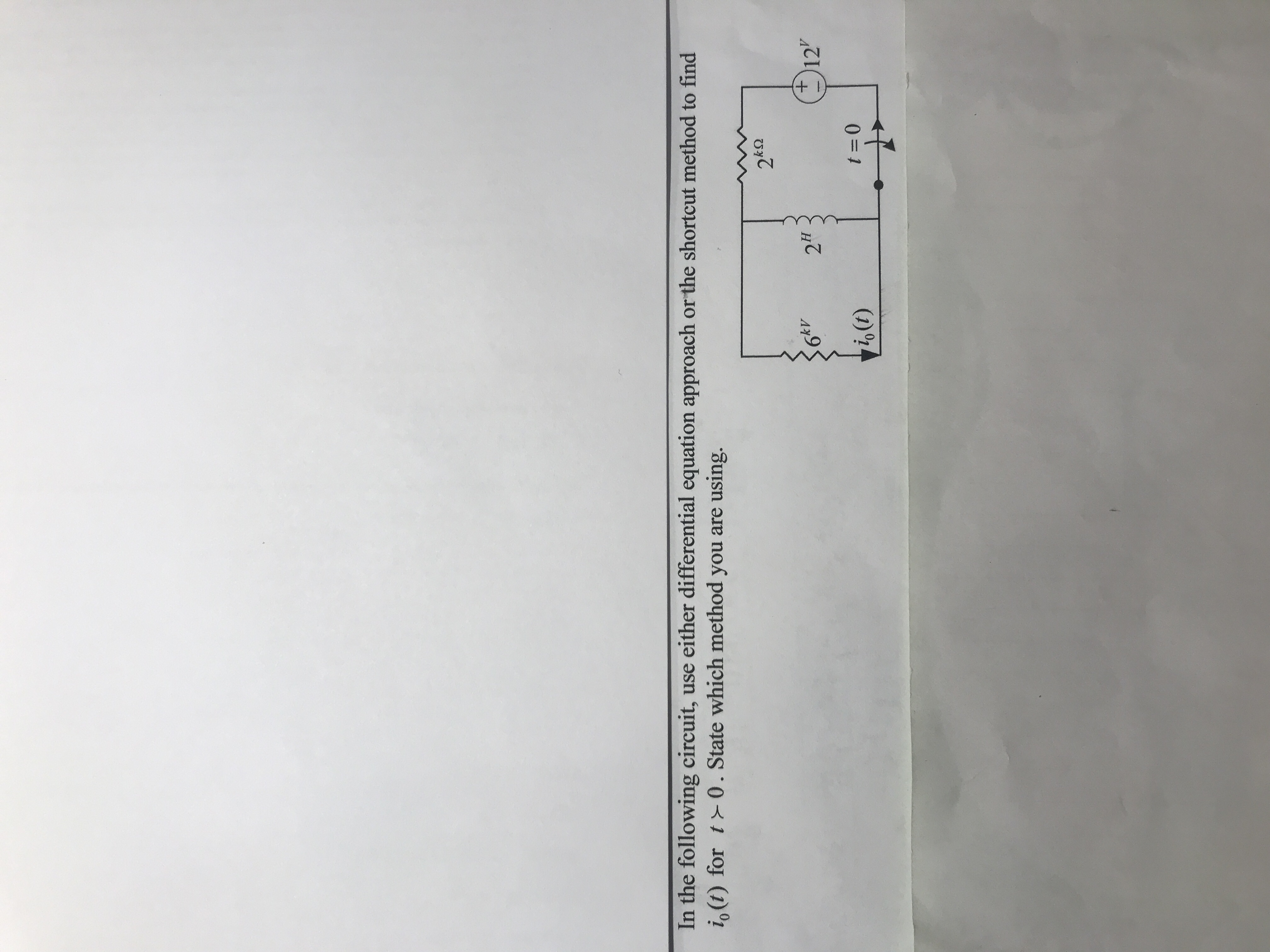# In the following circuit, use either differential equation approach or the shortcut method to findio(t) fort 0. State which method you are using.62"kV12o (t)t 0

Question
211 viewshelp_outlineImage TranscriptioncloseIn the following circuit, use either differential equation approach or the shortcut method to find io(t) fort 0. State which method you are using. 62" kV 12 o (t) t 0 fullscreen
check_circle

star
star
star
star
star
1 Rating
Step 1

STEP BY STEP METHOD:

From the circuit, write the expression for io(t) as follows:

Step 2

Here,

io(∞) is the current through the required branch (6 kilo ohms branch) at final state,

io(0+) is current through the required branch for t =0+, and

τ is the time constant for t >0.

From the given circuit, the switch opens at t =0. That is, switch is in closed condition before t =0. The inductor gets short circuited as the switch was closed for a long time. Therefore, redraw the circuit for t =0- as shown in Figure 1 and find the value of current through the inductor for t =0-.

Step 3

From Figure, it is clear that, the current in the circuit flows only through the short circuit path of ind...

### Want to see the full answer?

See Solution

#### Want to see this answer and more?

Solutions are written by subject experts who are available 24/7. Questions are typically answered within 1 hour.*

See Solution
*Response times may vary by subject and question.
Tagged in

### Electrical Engineering